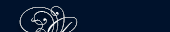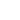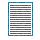НОВОСТИ   БИБЛИОТЕКА   ЭНЦИКЛОПЕДИЯ   КАРТА САЙТА   ССЫЛКИ   О САЙТЕ### Theorems

A theorem is a statement of a geometrical truth which has to be proved from facts already proved or assumed. The parts of a theorem are:

1) the General Enunciation - this states in general terms the truth which has to be proved.

2) the Particular Enunciation - this restates with reference to a particular figure the truth which has to be proved. It has two parts;

a) a statement of what is given and

b) a statement of what has to be proved.

3) The Construction - this states any lines or figures which are required for the proof.

4) The Proof - this proves the truth by facts already established or assumed.

The converse of a theorem proves what the theorem assumes and assumes what the theorem proves.

Example:

Theorem: If a triangle is equilateral, it is equiangular.

Converse: If a triangle is equiangular, it is equilateral.

If a theorem is true, it must not be assumed that its converse must be true. For example, consider the theorem: if two straight lines are parallel, they lie in the same plane. The converse of this is: if two straight lines are in the same plane, they are parallel, which is not true. Hence the converse of a theorem must -be proved separately.

Converse обратная теорема

enunciation формулировка

equiangular равноугольный

equilateral равносторонний

proof доказательство

prove доказывать

theorem теоремаПОИСК: